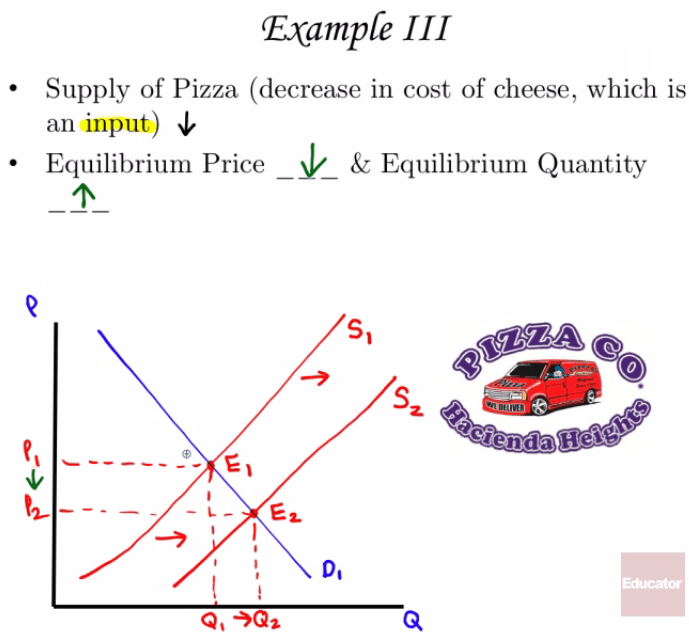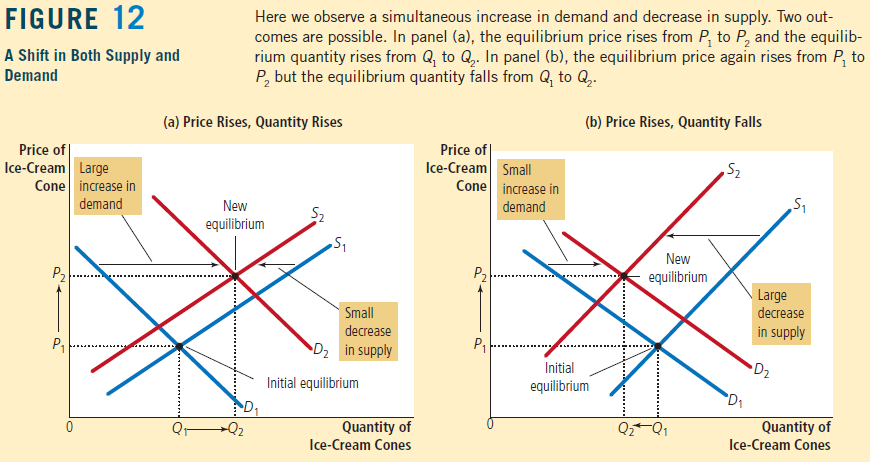Shifts of the Demand or Supply Curve

• Demand shift to the right

• Increase in equilibrium price

• Increase in equilibrium quantity

• Demand shift to the left

• Decrease in equilibrium price

• Decrease in equilibrium quantity

• Supply shift to the right

• Decrease in equilibrium price

• Increase in equilibrium quantity

• Supply shift to the left

• Increase in equilibrium price

• Decrease in equilibrium quantity

• Graph• ExamplesShifts of Both the Demand and Supply Curves

• Demand & supply shift to the right

• Increase in equilibrium quantity

• Equilibrium price unsure

• Demand & supply shift to the left

• Decrease in equilibrium quantity

• Equilibrium price unsure

• Supply shift to the left and demand shift to the right

• Increase in equilibrium price

• Equilibrium quantity unsure

• Supply shift to the right and demand shift to the left

• Decrease in equilibrium price

• Equilibrium quantity unsure

• Graph• Summary

• Change in same direction: equilibrium quantity for sure

• Change in opposite direction: equilibrium price for sure

• ExamplesChanges in equilibrium